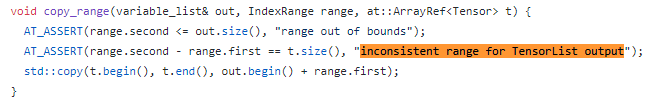# RuntimeError: inconsistent range for TensorList output

Hi all,

After upgrading to v0.4, when I reran my previous code which could be successfully run on v0.3.1,
I got the error message as shown in the figure:

And I found this error message comes from “pytorch/tools/autograd/templates/Functions.cpp”:(Error message happened while executing the “optimizer.backward()” function)

At first, I thought that there may be some mistakes in my code, so I checked it again and again, and finally got still the same error messageHowever, I found that if I downgrade from v0.4 to v0.3.1, this error suddenly disappeared and the code works as it should be…

Is there anyone knows what happened to this error @@?

Thanks!!!

Could you provide a small code sample reproducing this error in `0.4.0`?

In fact, I think this error will appear in the CycleGAN code since my training structure is similar to it.
(I am now updating the old v0.3.1’s official CycleGAN code to v0.4 to test.)

Here are the optimizers:

``````optimizer_g = torch.optim.Adam(itertools.chain(netGX.parameters(), netGY.parameters()), lr=g_lr, betas=[beta1, beta2])
optimizer_dx = torch.optim.Adam(netDX.parameters(), lr=d_lr, betas=[beta1, beta2])
optimizer_dy = torch.optim.Adam(netDY.parameters(), lr=d_lr, betas=[beta1, beta2])
``````

And the training part here:

``````    for i, (real_X, real_Y) in enumerate(zip(tr_x_loader, tr_y_loader)):
if (i+1) % log_step == 1:
iter_time = time.time()

# input image data
real_X = real_X.to(device)
real_Y = real_Y.to(device)

# freeze discriminators

# train generator netGX ---------------------------------------------------------------------
fake_Y = netGX(real_X)
DY_fake_decision = netDY(fake_Y)
GX_loss = MLE_loss(fake_Y, DY_fake_decision)

G_loss = GX_loss # + GY_loss + CX_loss + CY_loss

G_loss.backward()
optimizer_g.step()

# ...
``````

The error cames from this line of code:

``````G_loss.backward()
``````

And the functions “MLE_loss” and “switch_gradient” are shown as follows:

``````def MLE_loss(x, pred):
``````
``````def switch_gradient(net_list, mode='off'):
if mode == 'off': # turn off the gradients
for net_id, net in enumerate(net_list):
for p in net.parameters():
else: # turn on the gradients
for net_id, net in enumerate(net_list):
for p in net.parameters():
``````

However, if I change this line of code:

``````GX_loss = MLE_loss(fake_Y, DY_fake_decision)
``````

to

``````GX_loss = MLE_loss(fake_Y, DY_fake_decision.detach())
``````

then the error disappeared, but the results are wrong.

Hi,

I found out that if I comment out this line of code:

``````switch_gradient([netDX, netDY], mode='off')
``````

then everything will be fine…

But this line of code could work on v0.3.1…

Does anyone know what happened to this problem?

Thanks!!!